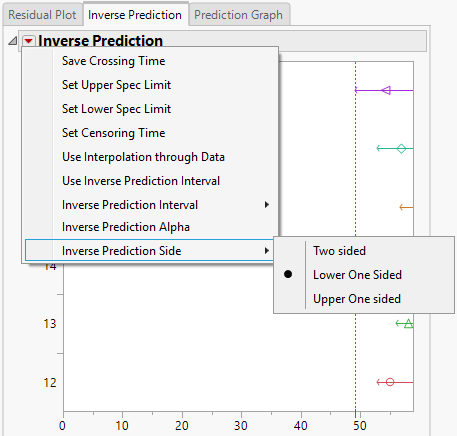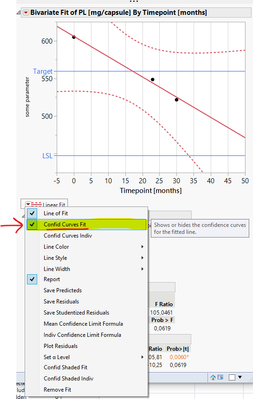Choose Language Hide Translation Bar
Highlighted

## One sided confidence interval in stability plots

Working on stability of pharmaceuticals, I was wondering whether the stability script (Analyze -> Reliability and Survival -> Degradation -> Stability Test) allows estimation of shelf life based on a one sided confidence interval. The default script, as it is set up now, is exclusively based on the intersection between a user defined acceptance limit and the two-sided 95% confidence interval. However, ICH Q1E suggests the use of a one-sided confidence interval for attributes known in- or decrease over time.

Since I can't script (yet) I wondered whether:

- there is a quick fix,

- there exists a justification to not use one-sided confidence intervals for attributes that either in- or decrease

- SAS / JMP could modify the stability script to allow the user to select one sided confidence intervals (both upper & lower).

I am sure that this is a significant issue in the pharmaceutical industry.

2 ACCEPTED SOLUTIONS

Accepted Solutions
Highlighted

## Re: One sided confidence interval in stability plots

Here is the menu we are discussing:Only the Lower One Sided option is available when you use the specialized Stability Test launch tab. The other options are available if you use one of the other two launch tabs.

Learn it once, use it forever!
Highlighted

## Re: One sided confidence interval in stability plots

My bad. The following comments after feedback from technical support and some research on my own:

• Fit Y by X uses two-sided confidence intervals. Setting alpha to 0.10 there will give confidence intervals identical to the (default) confidence intervals plotted by the stability module.

• The stability module uses one-sided confidence intervals. An alpha of 0.05 (the default value under prediction settings which can't be changed by the user it seems) will plot confidence intervals identical to an alpha of 0.10 in the Fit Y by X module. The stability module will however also plot the confidence interval on both sides of the curve.

My principal misunderstanding was to assume that a one-sided confidence interval must extend beyond a two-sided confidence interval but in fact the opposite is true. What the stability module does is it uses a two-sided (alpha = 0.10) confidence interval as one-sided (alpha = 0.05) confidence interval. I suspect that under the assumption of normality this is only an approximation?

I.e.: the 95% two-sided confidence interval of a normal distribution is given by +/-1.96 standard deviations (47.5% above the mean and 47.5% below the mean). If one wants to determine a one-sided confidence interval the ratio of the proportions above over below the mean (or vice versa) then changes to 50% (or infinite standard deviations really) over 45%. These 45% coverage on side (i.e. 95% in total) should correspond with 1.64 standard deviations. So, it is not clear to me why JMP makes the above approximations. What is clear to me however is that they are done and how they are done.

If I haven't yet reached the limit of your patience yet please let me know if any of the above is so wildly inaccurate that it should be removed from the user forum.

(There seems to be a problem with posting stuff to the forum at the moment, so please excuse if at some point this reply comes up multiple times!)

10 REPLIES 10
Highlighted

## Re: One sided confidence interval in stability plots

You are using the correct platform. The default is lower one-sided intervals. Select the Inverse Prediction tab. Click the red triangle next to Inverse Prediction and select Inverse Prediction Side to see which type of interval is currenlty reported. Mine shows lower one-sided intervals.

Learn it once, use it forever!
Highlighted

## Re: One sided confidence interval in stability plots

Thank you. That is helpful. Now how do I actually change the inverse predicition side, i.e. is it possible to toggle between one- and two-sided confidence intervals? When I try changing the setting it won't. It just stays where it is. Is this a default setting, that the software will pick whatever confidence interval is most adequate for my data set?

Highlighted

## Re: One sided confidence interval in stability plots

Here is the menu we are discussing:Only the Lower One Sided option is available when you use the specialized Stability Test launch tab. The other options are available if you use one of the other two launch tabs.

Learn it once, use it forever!
Highlighted

## Re: One sided confidence interval in stability plots

Thank you very much again. I found the menu, didn't dare to work in one of the other two tabs so far. Will check it out asap.

Highlighted

## Re: One sided confidence interval in stability plots

Hi, I am returning with a follow-up question. I hope you are well these days and that none of your family, friends or colleagues area affected by SARS-CoV-2.

Today i analyzed a miniature dataset both using "Fit Y by X" and the stability modules. As there are differences between these approaches I was expecting different x-values (timepoints) for when the confidence interval crosses the lower acceptance limit. What confused me was that the stability module gave a longer shelf-life estimate, although I believed that using a two sided confidence interval would actually give shorter shelf-life estimate, compared to the "Fit Y by X" estimate. What am I missing? I have attached a picture of the two results for explanation. Thank you very much for an answer in advance.

Highlighted

## Re: One sided confidence interval in stability plots

Which type of interval are you plotting with Bivariate? Confidence or prediction?

Learn it once, use it forever!
Highlighted

## Re: One sided confidence interval in stability plots

I used what's shown in the screenshot below with alpha = 0.05. Thanks for this quick response!Highlighted

## Re: One sided confidence interval in stability plots

I am out of ideas then. I recommend that you contact JMP Technical Support (support@jmp.com) with this question and report back here with your solution.

Learn it once, use it forever!
Highlighted

## Re: One sided confidence interval in stability plots

My bad. The following comments after feedback from technical support and some research on my own:

• Fit Y by X uses two-sided confidence intervals. Setting alpha to 0.10 there will give confidence intervals identical to the (default) confidence intervals plotted by the stability module.

• The stability module uses one-sided confidence intervals. An alpha of 0.05 (the default value under prediction settings which can't be changed by the user it seems) will plot confidence intervals identical to an alpha of 0.10 in the Fit Y by X module. The stability module will however also plot the confidence interval on both sides of the curve.

My principal misunderstanding was to assume that a one-sided confidence interval must extend beyond a two-sided confidence interval but in fact the opposite is true. What the stability module does is it uses a two-sided (alpha = 0.10) confidence interval as one-sided (alpha = 0.05) confidence interval. I suspect that under the assumption of normality this is only an approximation?

I.e.: the 95% two-sided confidence interval of a normal distribution is given by +/-1.96 standard deviations (47.5% above the mean and 47.5% below the mean). If one wants to determine a one-sided confidence interval the ratio of the proportions above over below the mean (or vice versa) then changes to 50% (or infinite standard deviations really) over 45%. These 45% coverage on side (i.e. 95% in total) should correspond with 1.64 standard deviations. So, it is not clear to me why JMP makes the above approximations. What is clear to me however is that they are done and how they are done.

If I haven't yet reached the limit of your patience yet please let me know if any of the above is so wildly inaccurate that it should be removed from the user forum.

(There seems to be a problem with posting stuff to the forum at the moment, so please excuse if at some point this reply comes up multiple times!)

Article Labels

There are no labels assigned to this post.